# The Cantellated 24-cell

The cantellated 24-cell is a uniform polytope bounded by 144 cells (24 rhombicuboctahedra, 24 cuboctahedra, 96 triangular prisms), 720 polygons (288 triangles, 432 squares), 864 edges, and 288 vertices.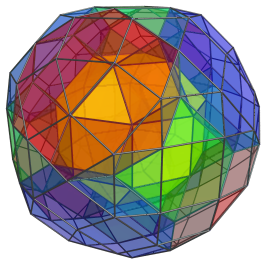## Structure

We shall explore the structure of the cantellated 24-cell by means of its parallel projection into 3D, centered on a rhombicuboctahedral cell.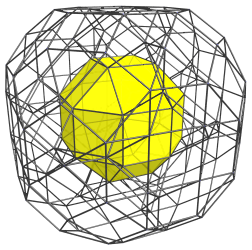The above image shows the nearest rhombicuboctahedron to the 4D viewpoint. Twelve of its square faces are joined to 12 triangular prisms, as shown below: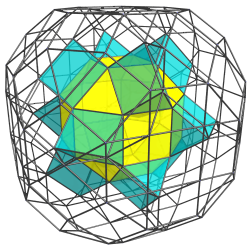The remaining 6 square faces of the rhombicuboctahedron are joined to 6 cuboctahedra: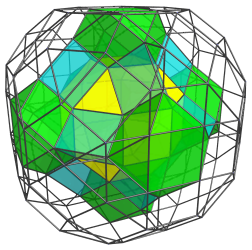These cuboctahedra are slightly flattened by the projection because they lie at a 45° angle to the 4D viewpoint, but in 4D they are perfectly uniform cuboctahedra.

The remaining 8 triangular faces of the nearest rhombicuboctahedron are joined to 8 other rhombicuboctahedra, as shown next as two groups of 4: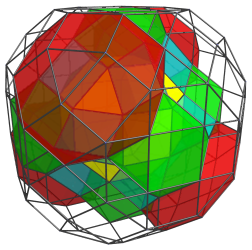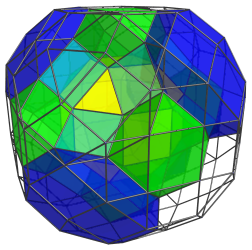Here are all of them together: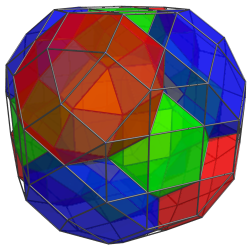There are 24 grooves into which 24 triangular prisms may be fitted: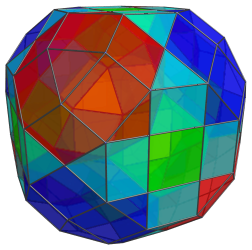These are all the cells that lie on the “Northern Hemisphere” of the cantellated 24-cell.

On the “equator” of the cantellated 24-cell are 6 rhombicuboctahedra, as shown below: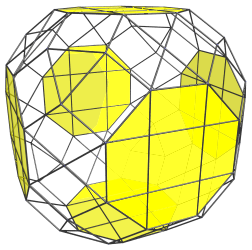These rhombicuboctahedra have been foreshortened into octagons, because they are seen at a 90° angle.

There are also 12 cuboctahedra on the equator, shown next: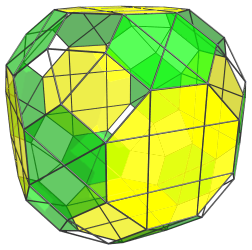Lastly, there are 24 equatorial triangular prisms: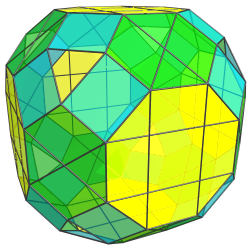These triangular prisms have been foreshortened into rectangles because they are seen at a 90° angle from the 4D viewpoint. The edges of the rectangles that touch the octagons are actually foreshortened square faces that the triangular prisms share with the rhombicuboctahedra.

The 8 remaining triangular gaps are not images of any equatorial cells; they are the triangular faces where the 8 rhombicuboctahedra in the “northern hemisphere” touch their counterparts in the “southern hemisphere”. The layout of cells in the southern hemisphere exactly mirrors the layout in the northern hemisphere, with the layers reversed.

In summary, the northern hemisphere has 1+8=9 rhombicuboctahedra, 6 cuboctahedra, and 12+24=36 triangular prisms. The equator has 6 rhombicuboctahedra, 12 cuboctahedra, and 24 triangular prisms. The southern hemisphere has the same counts as the northern hemisphere: 9 rhombicuboctahedra, 6 cuboctahedra, and 36 triangular prisms. In total, therefore, there are 9+6+9=24 rhombicuboctahedra, 6+12+6=24 cuboctahedra, and 36+24+36=96 triangular prisms.

## Perspective Projections

The following image shows the perspective projection of the cantellated 24-cell from the same viewpoint as the previous section.We use the same coloring scheme as we used in displaying the northern hemisphere cells, except with the colors made more transparent so that the deep structure of the projection is visible.

## Coordinates

The Cartesian coordinates of the cantellated 24-cell, centered on the origin and having edge length 2, are all permutations of coordinates and changes of sign of:

• (0, √2, √2, 2+2√2)
• (1, 1+√2, 1+√2, 1+2√2)

The second set of coordinates corresponds with the vertices of a runcitruncated tesseract, which can be inscribed inside the cantellated 24-cell.

Last updated 17 Jun 2019.